#### Theory of Non-Linearity in Transducers

Introduction:

Apart from nominal manufacturing variations and temperature results on the performance of transducer, there are other factors that cause errors in the measurement and limit the accuracy that can be obtained. Transducers seldom possess a perfectly linear transfer characteristic, however always has certain degree of non-linearity over their range of operation and diverge from the ideal straight-line output voltage vs. input pressure relationship as shown in figure below. Second order non-linearity in the characteristic generally limits the error to one direction: either pressure will be slightly under-estimated or slightly over-estimated, depending on the slope of curve. Third order non-linearity signifies that the slope of the characteristic can change direction across the range of operation and hence the pressure might be underestimated in one portion of the range and overestimated in the other portion.

Moreover properties like hysteresis and ageing effects can as well give mount to measurement errors. Hysteresis means that the transducer might give a slightly distinct output voltage for similar value of applied pressure depending on whether the pressure is rising or decreasing. Such sources of error are generally quantified by manufacturers in data sheets for the devices as shown in table below.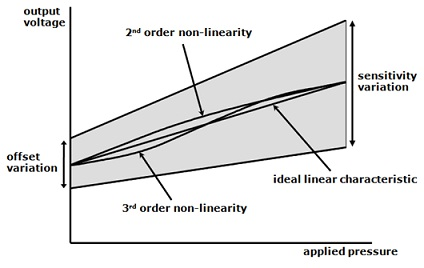Figure: Transfer Characteristic of the SX 50 Pressure Transducer

Table: The Performance Parameters of the SX 50 Pressure Transducer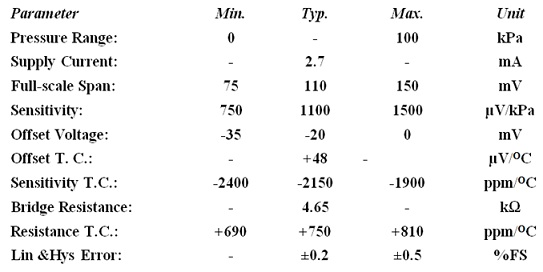Non-linearity: Static Analysis

A transfer characteristic containing second order non-linearity can be explained by a quadratic in the applied pressure as:

Vout = a + bp + cp2

Where the coefficients are generic and explain the degree of non-linearity present. When a two point calibration technique is used then the conditioning amplifier will be adjusted to provide an output of 0V for an input applied pressure of zero. When this is the case then the coefficient ‘a’ is efficiently adjusted to zero and hence the amplifier output becomes:

Vout = bp + cp2

The coefficient ‘c’ will usually be much smaller than ‘b’ as merely a small degree of non-linearity must be present. Ideally the transducer output must be purely linear and determined by the bridge supply voltage and the span sensitivity of transducer in such a way that:

Vout = SVBp

Error in the output voltage can then be explained by the difference between the real and the ideal characteristics:

ε = bp + cp2 - SVBp

If a two-point calibration process is employed so that the amplifier gain or the bridge supply voltage is adjusted to give the necessary maximum output voltage at full-scale pressure input, pmax. Then the error can be taken as zero (0) for maximum input pressure and hence:

bpmax + cpmax2 - SVBpmax = 0

cpmax2 = (SVB - b) pmax

And hence,

C = (SVB - b)/ pmax

The error can then be explained by:

ε = bp + [(SVB - b)/ pmax] p2 - SVBp

The pressure at which maximum error takes place can be found by taking the derivative as:

dε/dp = b + [2(SVB - b)/ pmax] p - SVB = 0

That gives,

[2(SVB - b)/ pmax] p = SVB - b

And hence,

p = [(SVB - b)/ pmax]/2(SVB - b) = pmax/2

This exhibits that the maximum error caused by non-linearity takes place at half full-scale. The value of this error is as follows: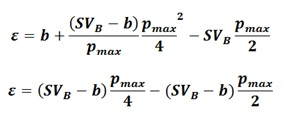And hence,

ε = - (SVB - b) (pmax/4)

Whether this error is negative or positive depends on whether the coefficient ‘b’ is greater than or less than SVB.

This error is the worst-case value for second order non-linearity. It can thus be used to point out the maximum error caused by the non-linearity in transducer. This is completed by expressing its magnitude as a fraction or percentage of ideal full scale output voltage. This provides: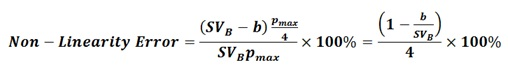Non-linearity: Simple Dynamic Input

The problem with non-linearity is obvious whenever a time varying input is included. Therefore, when monitoring a pressure that differs with time, the amplifier will produce an electrical output signal that is a function of time. This is the subject to nonlinearity of transducer characteristic and hence:

Vout(t) = bp(t) + cp(t)2

If, for illustration, the input pressure is taken as being sinusoidal in nature as is explained as:

p(t) = pm cos (ωt)

Then the output voltage is as follows:

p(t) = b pm (ωt) + cp2m cos2 (ωt)

By using the trigonometric identity cos2A= 1/2[1+cos (2ωt)] gives:

p(t) = b pm (ωt) + 0.5 cp2m cos (2ωt)

Note that the initial term in this expression is scaled, presumably amplified, version of the input pressure. The second term symbolizes a dc component that is produced at the output however is not present at the input. This is essentially an offset voltage that is produced solely as an outcome of the non-linearity of transducer. The third term is a term with a time profile twice the frequency of the input signal, that is, it is a second harmonic of basic component that has a radian frequency ω. This leads to second harmonic distortion of the profile of original pressure signal whenever observed at the output of conditioning amplifier.

Non-linearity: Composite Input

Let consider a second input pressure situation where the input comprises of two frequency components:

p(t) = pm1 cos (ω1t) + pm2 cos (ω2t)

Then in this situation the output voltage from amplifier will be explained by: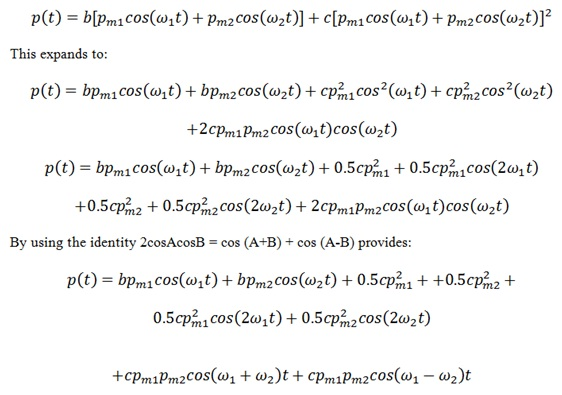The terms on left hand side on the first line are the scaled input components.

The terms on right hand side of the first line are dc offset components, with an offset contributed by each and every component of the input pressure.

The terms on second line are second harmonic components of each of the input frequencies existed.

At last the terms on last line are the sum and difference components of input frequencies. These are termed to as intermodulation components as they are not harmonically associated to the frequencies exist in the input applied pressure profile. Since of the lack of harmonic quality they frequently outcome in more severe distortion than that caused by the harmonic components. In speech, for illustration, they would have a more detrimental consequence on intelligibility than the harmonic components.

Let consider ω1 = 100Hz and ω2 = 80Hz

Then ω1 + ω2 = 180Hz and ω1 - ω2 = 20Hz

The sum component at 180Hz is greater than the highest input frequency and therefore could perhaps be filtered out by using a low-pass filter.

The difference component is lower than the lowest input frequency and therefore can’t be eliminated by low-pass filtering. High-pass filtering might appear to be an option however this would not be fitting if there were a dc or steady-state component in input applied pressure. The difference component is at a much low frequency and this can cause a low drift or wander-like modulation of the output signal. Usually little can be done in analogue circuitry to counteract the consequences of transducer non-linearity. It is usually a matter of deciding on the degree of non-linearity error which can be accepted and selecting an appropriate transducer accordingly.

With more expenditure, analogue-to-digital transformation can be employed in conjunction with a look-up table to correct non-linearity, as a rule for the nominal operating temperature. The look-up table stores a corrected value of the transducer reading that is accessed based on its real reading and a prior measure of the non-linearity of its transfer feature.

Design Example:

An illustration of a pressure monitor employed for blood pressure measurement is as shown in figure below. This circuit comprises full temperature compensation of both offset and span sensitivity. This has a two-point calibration that can be carried out in a single iteration of temperature cycle. It gives a digital readout of pressure however doesn’t comprise non-linearity correction.

The circuit is powered from a battery that feeds a 5V regulator IC1, with a low dropout voltage. The buffer op-amp IC6 is employed to produce a -0.5V secondary supply voltage to feed the other op-amps IC4 and IC5 that can’t operate from ±5V.The heart of electronic manometer is the digital panel meter (DPM), IC7, a MAX138 (Maxim Inc.), that is a differential-input, ratio metric DPM, containing a very high input common-mode-rejection-ratio, high temperature-stable performance, and also an on-chip positive-to-negative supply converter. This gives a direct drive facility for a 3½ digit liquid crystal display (LCD). The numerical output displayed on LCD is as follows:

N = 1000 x (VIN HI – VIN LO)/(VREF HI – VREF LO)

The reference voltage is received through a potential divider, R16, R17 and RV5, placed across an internal precision voltage reference that exists among the supply rail and the analogue common pin on DPM, IC7 pin 32. The potentiometer RV5 is employed to adjust the nominal full-scale value on the display at lower calibration temperature. The other ancillary components joined to IC7 are chosen in accordance with the manufacturer's recommendations for the optimum performance.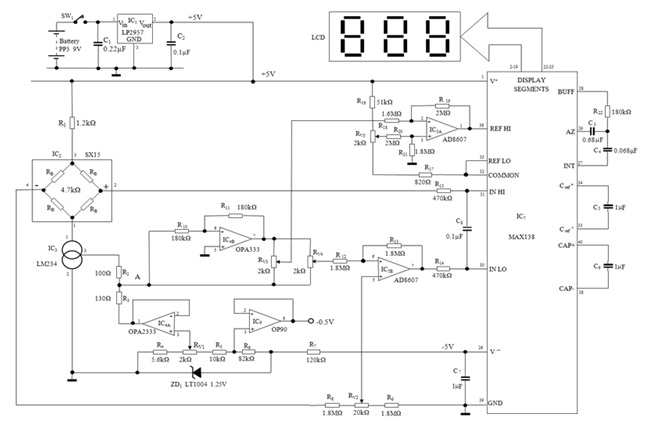Figure: Schematic Diagram of Pressure Transducer for monitoring Blood Pressure

The SX15 transducer, IC2, is driven by programmable current source, IC3, value of current being set by resistors R2, R3 and the voltage at the output pin 1 of op-amp IC4A. Such components are as well employed to match the temperature coefficient of the current source to that of transducer in order to give nominal temperature compensation of the full-scale span over working range. IC4 is selected to have a very low temperature coefficient of offset voltage and hence the voltage at pin 1 is essentially temperature-independent. The input potential to IC4A that acts as a buffer amplifier is given by the potential divider comprising of the band gap reference diode, ZD1, containing a very low temperature coefficient, resistors R4 – R7 and RV1 that is employed to adjust it. The positive output voltage from pin 2 of transducer, IC2, is fed through one side of the low-pass filter consisting of R15 and C8 to positive differential input pin, IN HI, of the panel meter, IC7 pin 31. The output voltage from the negative side of transducer, IC2 pin 4, is scaled by a factor of ½ by the potential divider comprising of R8, R9 and RV2, however is subsequently provided a gain of 2 in the summing amplifier composed of IC5B, R12 and R13. The potentiometer RV2 is employed to cancel the nominal offset voltage of transducer at lower calibration temperature. The output of amplifier, IC5B pin 7, is fed into the negative differential input of the panel meter, IN LO, IC7 pin 30, through the other side of the low-pass filter comprised of R14 and C8. This makes sure that only slowly changing pressure is measured and displayed on LCD.

The output voltage at pin 3 of programmable current source, IC3, has accurate and linear temperature dependence however not zero at room temperature is. The resistors R2 and R3 are employed to modify the efficient temperature coefficient of current source by the ratio of their values and hence it closely matches the nominal value of transducer temperature coefficient. The negative potential at output of IC4A is then employed to obtain a zero potential at node A at lower calibration temperature, which then rises linearly above this temperature. This potential is fed to one side of two potentiometers, RV3 and RV4 and as well to the inverting amplifier comprising of IC4B and resistors R10 and R11 to produce an equivalent negative temperature dependent voltage that is then fed to other side of the potentiometers. With IC4 selected for a very low temperature coefficient of offset voltage, this gives perfectly balanced positive and negative temperature dependent voltages on each and every side of the potentiometers. This mechanism as well signifies that, regardless of the setting of RV3 and RV4, the potentials at wipers of both of such potentiometers are zero at lower calibration temperature as needed for a single cycle calibration process. The potential at output of RV4 is added up to the differential voltage of the transducer in amplifier comprising of IC5B and resistors R12 and R13. This is then adjusted to accurate the temperature coefficient of the offset voltage of transducer at upper calibration temperature. Potential at the output of RV3 is then added to the nominal reference voltage of DPM in the amplifier IC5A and resistors R18 – R21. This is adjusted to counteract the temperature coefficient of transducer sensitivity at the upper calibration temperature.The following calibration process is employed:

A) Initially all the potentiometers are centred.

B) The oven temperature is fetched to 20oC.

C) RV1 is adjusted to provide 0V at node A.

D) Input pressure of 0 mm Hg is applied.

E) RV2 is adjusted till the LCD exhibits ''000''.

F) The input pressure is raised to 300 mmHg.

G) RV5 is adjusted till LCD reads ''300''.
H) The oven temperature is then increased to 40o C.

I) Input pressure is set to 0 mmHg again.

J) RV4 is adjusted till the LCD reading is ''000''.

K) Input pressure is increased to 300 mmHg.

L) RV3 is adjusted till the LCD reading is ''300''.

Latest technology based Electrical Engineering Online Tutoring Assistance

Tutors, at the www.tutorsglobe.com, take pledge to provide full satisfaction and assurance in Electrical Engineering help via online tutoring. Students are getting 100% satisfaction by online tutors across the globe. Here you can get homework help for Electrical Engineering, project ideas and tutorials. We provide email based Electrical Engineering help. You can join us to ask queries 24x7 with live, experienced and qualified online tutors specialized in Electrical Engineering. Through Online Tutoring, you would be able to complete your homework or assignments at your home. Tutors at the TutorsGlobe are committed to provide the best quality online tutoring assistance for Electrical Engineering Homework help and assignment help services. They use their experience, as they have solved thousands of the Electrical Engineering assignments, which may help you to solve your complex issues of Electrical Engineering. TutorsGlobe assure for the best quality compliance to your homework. Compromise with quality is not in our dictionary. If we feel that we are not able to provide the homework help as per the deadline or given instruction by the student, we refund the money of the student without any delay.

2015 ©TutorsGlobe All rights reserved. TutorsGlobe Rated 4.8/5 based on 34139 reviews.